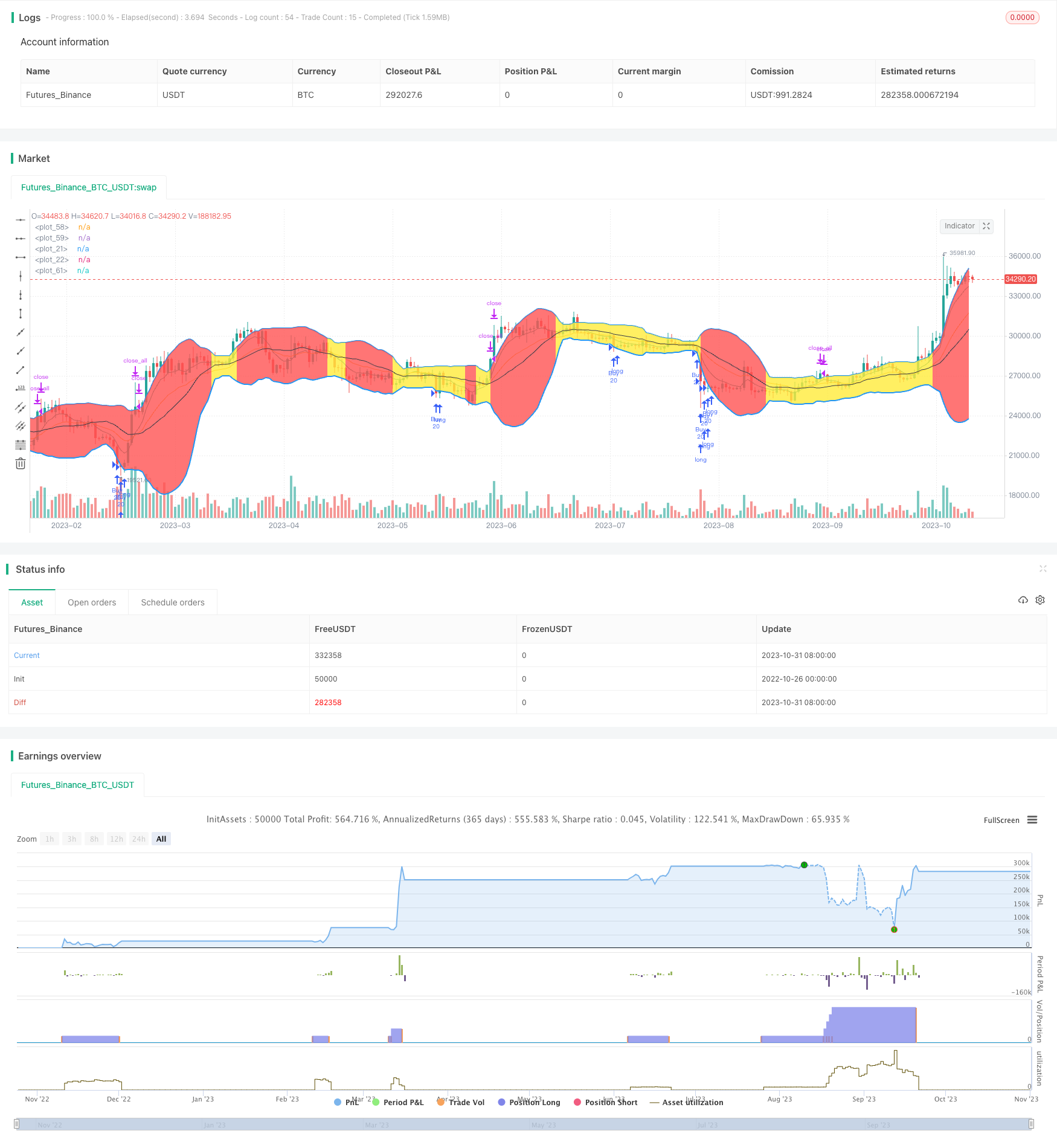# DAKELAX-XRPUSDT Bollinger Band Mean Reversion Strategy

Author: ChaoZhang, Date: 2023-11-02 16:18:34
Tags:## Overview

DAKELAX-XRPUSDT is a trading bot strategy for XRPUSDT on Binance. It is a simple reverse to mean strategy using Bollinger Bands, and performs well in backtest on H1 timeframe from May to Aug 2019, as well as running live.

## Strategy Logic

The strategy first calculates the 20-period SMA and upper/lower Bollinger Bands. The upper band is SMA + 1.5 standard deviation, and the lower band is SMA - 2.2 standard deviation. It then calculates the contraction rate of the bands. Bands are filled black if contraction > 1.3, yellow if < 0.1, else red.

When close price is below the lower band, it goes long with 20 coins. When close is above upper band, it closes all positions.

The strategy also calculates 7-period EMA fast line and 18-period EMA slow line. Crossover of fast line above slow line is buy signal, and crossover below is sell signal.

• Bollinger Bands and contraction rate intuitively identify trends and volatility
• Combine with EMA crossover adds strength to signals
• Good backtest results and relatively stable in live trading

## Risk Analysis

• High probability of failure when breakout after band contraction
• Too many crossovers in ranging markets risks losses
• Only considers daily factors, misses larger timeframe trends

Consider dynamic position sizing or stop loss to control risks. Optimize crossover strategy to avoid whipsaws in ranging markets. Add higher timeframe trend indicators to identify larger moves.

## Optimization Directions

• Adjust buy amount based on band width, less when contracted and more when expanded

• Consider accumulating positions when contraction seen but signal not triggered yet

• Add longer timeframe trend INDICATOR to determine overall direction, pause strategy when unclear

• Incorporate stop loss to control risk, can set near recent lows of bands

• Optimize crossover parameters like EMA periods to avoid getting trapped

## Summary

DAKELAX-XRPUSDT is a trading bot strategy using Bollinger Band contraction with EMA crossover. It is intuitive and has good backtest results but contains some risks. These can be reduced through position sizing, stopping strategy, adding stop loss and optimizing crossover logic. Overall it provides a clear example of a Bollinger Band strategy, but requires pair-specific optimization for stable live profits.

```/*backtest
start: 2022-10-26 00:00:00
end: 2023-11-01 00:00:00
period: 1d
basePeriod: 1h
exchanges: [{"eid":"Futures_Binance","currency":"BTC_USDT"}]
*/

//@version=3

// SMA20
len2 = input(20, minval=1)
src2 = input(close)
out2 = sma(src2, len2)

// BB contraction value (medium tight)
contraction_value = 1.3
// BB contraction value (very tight)
contraction_value2 = 0.1

// 2xSTDEV BB calculation
dev = stdev(src2, len2)
upper_BB = out2  + 1.5*dev
lower_BB = out2  - 2.2*dev
x1 = plot(upper_BB, color=blue, linewidth = 2)
x2 = plot(lower_BB, color=blue, linewidth = 2)

contraction = (upper_BB-lower_BB)/out2

//fills the BBands according to the contraction value (threshold)

// Calculate values
fastMA  = ema(close, 7)
slowMA  = ema(close, 18)

crossUp   = crossover(fastMA, slowMA)
crossDown = crossunder(fastMA, slowMA)

buySignal   = (crossUp or crossUp) and (low > slowMA)
shortSignal = (crossDown or crossDown) and (high < slowMA)

// Highlight alerts on the chart
bgColour =
(buySignal and barstate.isrealtime) ? green :
(shortSignal and barstate.isrealtime) ? red :
na

signalSell = (shortSignal ) ? true : false

test = true

test := not test

closesBelowLowerBB = close < lower_BB
closesAboveUpperBB = close > upper_BB

tmptext = "blah"

// Plot values
plot(series=fastMA, color=teal)
plot(series=slowMA, color=orange)

plot(out2, color=black, linewidth = 1)
fill(x1, x2, color = contraction > contraction_value ? black : contraction < contraction_value2 ? yellow: red)

isInRed = contraction < contraction_value and contraction >= contraction_value2
isInYellow = contraction < contraction_value and contraction < contraction_value2

if ( closesBelowLowerBB )